Courses

## 10 Questions MCQ Test Electronic Devices | Test: DC Load Lines

Description
This mock test of Test: DC Load Lines for Electrical Engineering (EE) helps you for every Electrical Engineering (EE) entrance exam. This contains 10 Multiple Choice Questions for Electrical Engineering (EE) Test: DC Load Lines (mcq) to study with solutions a complete question bank. The solved questions answers in this Test: DC Load Lines quiz give you a good mix of easy questions and tough questions. Electrical Engineering (EE) students definitely take this Test: DC Load Lines exercise for a better result in the exam. You can find other Test: DC Load Lines extra questions, long questions & short questions for Electrical Engineering (EE) on EduRev as well by searching above.
QUESTION: 1

### Which of the following depicts the DC load line?

Solution:

In transistor circuit analysis, sometimes it is required to know the collector currents for various collector emitter voltages. The one way is to draw its load line. We require the cut off and saturation points.

QUESTION: 2

### For the circuit shown, find the quiescent point.​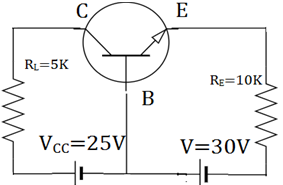Solution:

We know, IE=VEE/RE=30/10kΩ=3mA
IC=α IE =IE =3mA
VCB=VCC-ICRL=25-15=10V. So, quiescent point is (10V, 3mA).

QUESTION: 3

### Which of the following depicts the load line for the circuit shown below?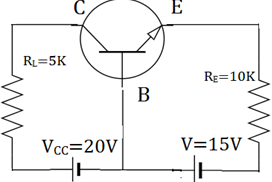Solution:

We know, IE=VEE/RE=15/5kΩ=3mA
IC=α IE =IE =3mA
VCB=VCC-ICRL=20-15=5V. So, quiescent point is (5V, 3mA).

QUESTION: 4

For the circuit shown, find the quiescent point.​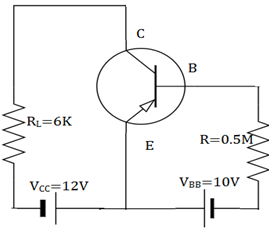Solution:

We know, VCE=12V
(IC)SAT =VCC/RL=12/6K=2mA. IB=10V/0.5M=20µA. IC= βIB=1mA. I
VCE=VCC-ICRL=12-1*6=6V. So, quiescent point is (6V, 1mA).

QUESTION: 5

Which of the following depicts the load line for the given circuit?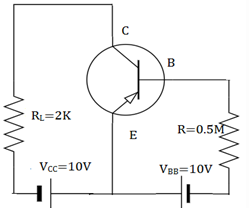Solution:

We know, VCE=6V
(IC)SAT =VCC/RL=10/2K=5mA. IB=10V/0.5M=20µA. IC= βIB=1mA. I
VCE=VCC-ICRL=10-1*2=8V. So, quiescent point is (8V, 1mA).

QUESTION: 6

The DC equivalent circuit for an NPN common base circuit is.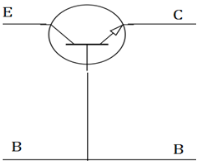Solution:

In the common base circuit, the emitter diode acts like a forward biased ideal diode, while collector diode acts as a current source due to transistor action. Thus an ideal transistor may be regarded as a rectifier diode in the emitter and a current source at collector.

QUESTION: 7

The DC equivalent circuit for an NPN common emitter circuit is.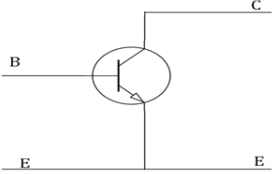Solution:

In the common emitter circuit, the ideal transistor may be regarded as a rectifier diode in the base circuit and a current source in the collector circuit. In the current source, the direction of arrow points in direction of conventional current.

QUESTION: 8

What is the other representation of the given PNP transistor connected in common emitter configuration?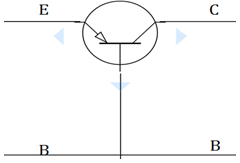Solution:

The emitter junction is forward biased with the help of battery VEE by which, negative of the battery is connected to the emitter while positive is connected to base. RE is the emitter resistance. The collector junction is reversed biased.

QUESTION: 9

What is the DC characteristic used to prove that the transistor is indeed biased in saturation mode?

Solution:

When in a transistor is driven into saturation, we use VCE(SAT) as another linear parameter. In, addition when a transistor is biased in saturation mode, we have IC < βIB. This characteristic used to prove that the transistor is indeed biased in saturation mode.

QUESTION: 10

For the circuit shown, find the quiescent point.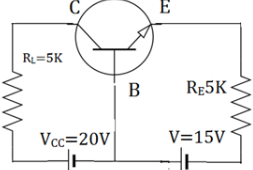Solution:

We know, IE=VEE/RE=10/5kΩ=2mA
IC=α IE =IE =2mA
VCB=VCC-ICRL=20-10=10V. So, quiescent point is (10V, 2mA).